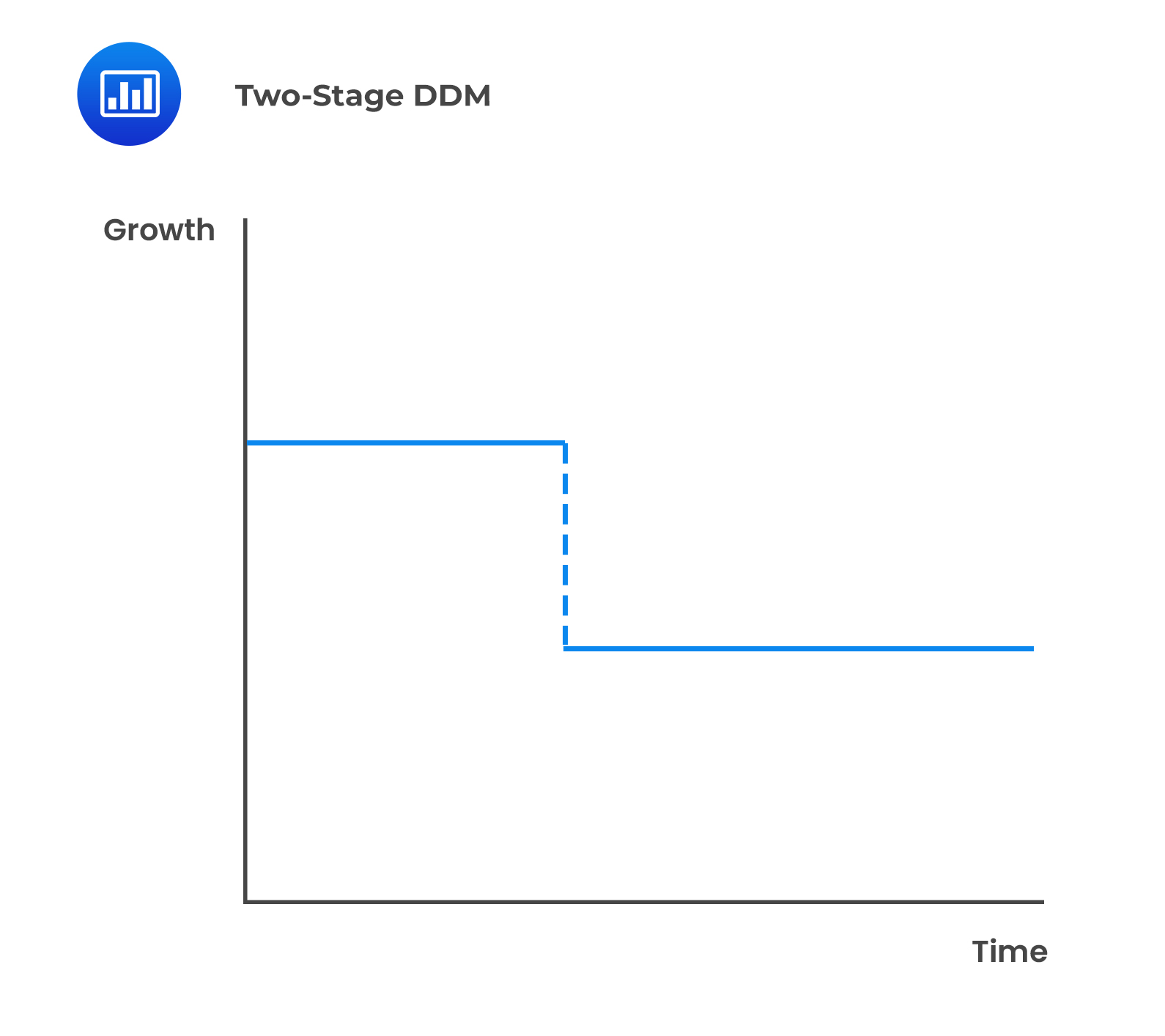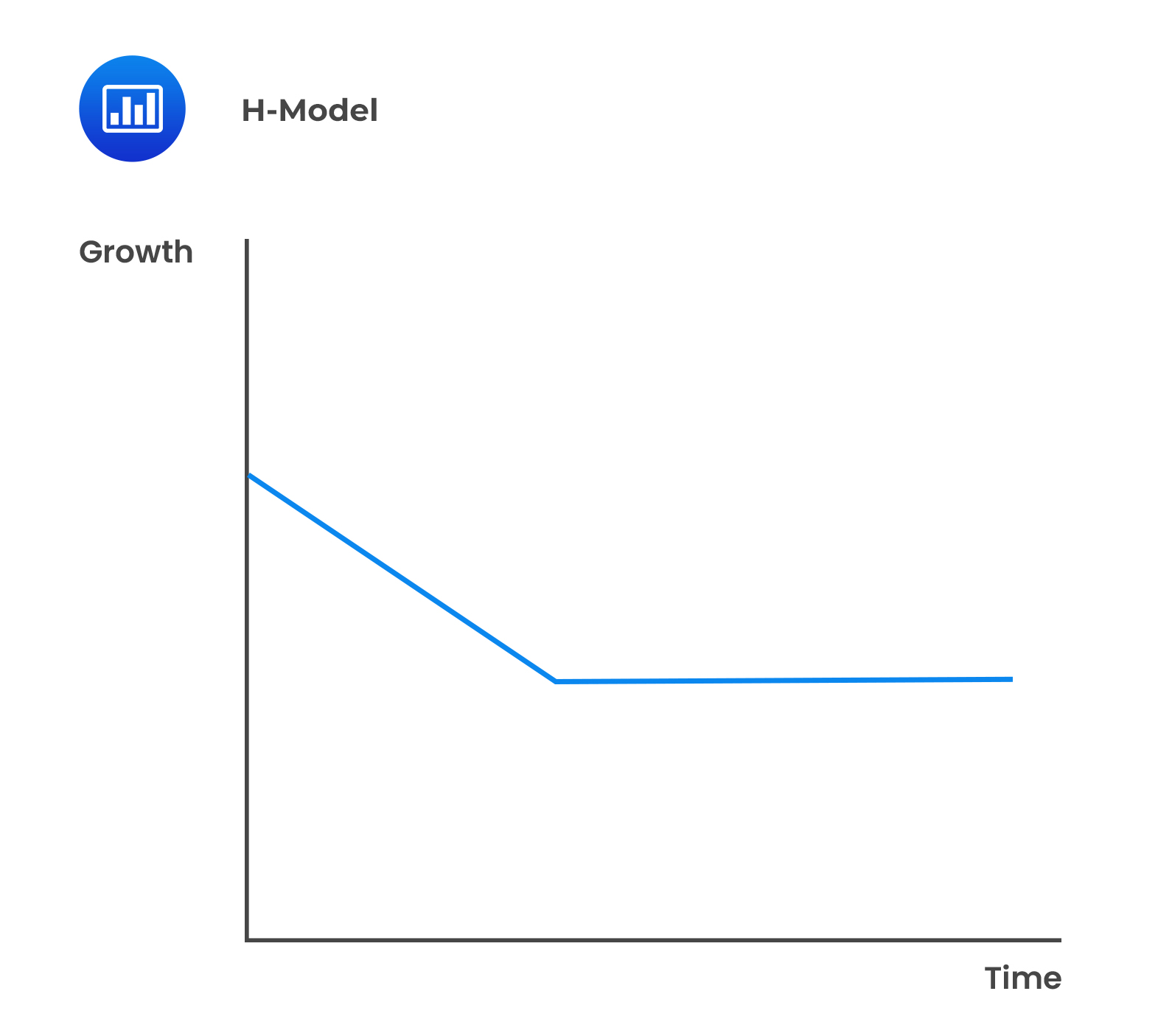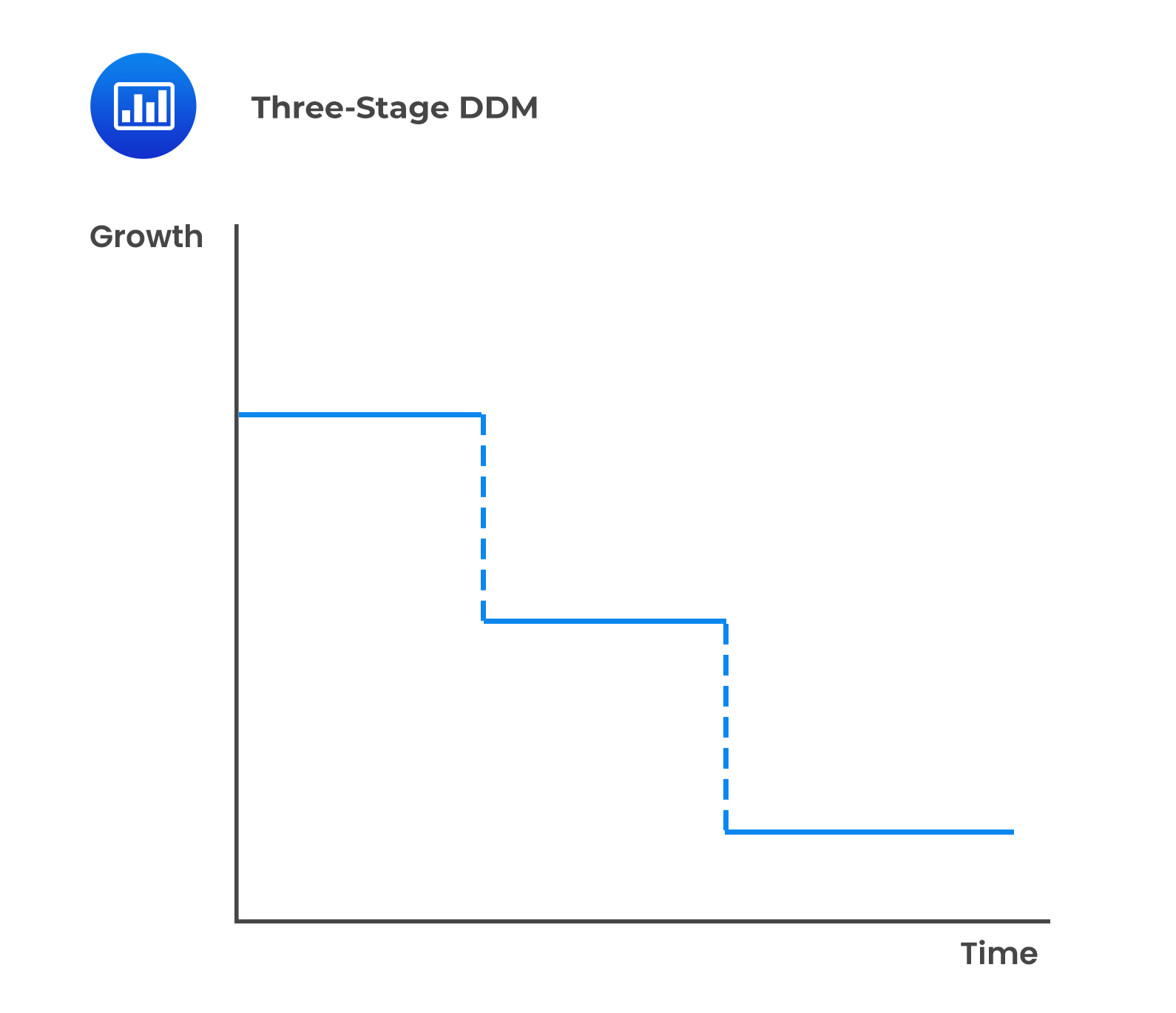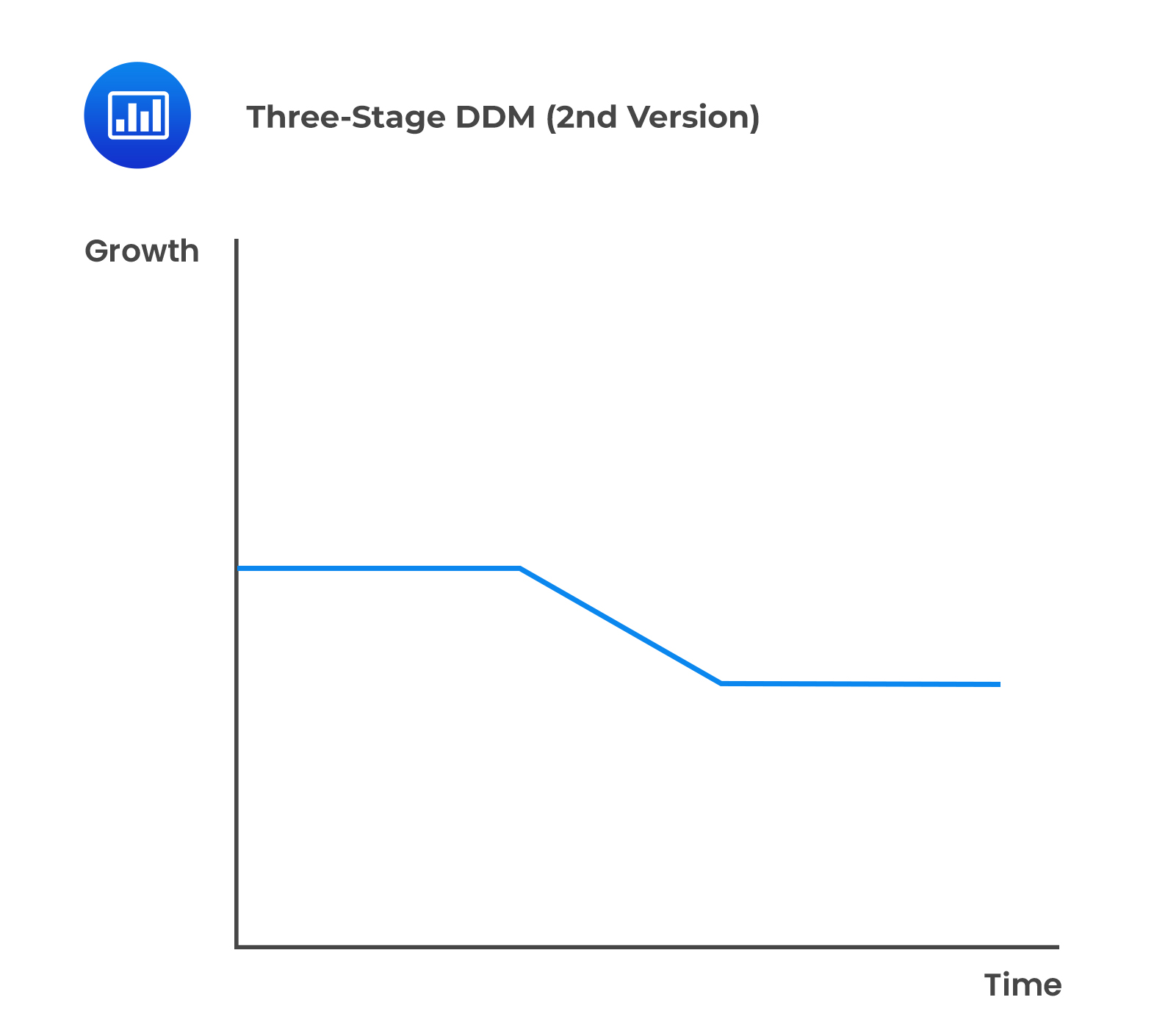# Multiperiod Models

## Two-Stage DDM

There are several assumptions of the first version of the two-stage DDM:

• The first stage represents a period of abnormal growth.
• The second stage represents a period of sustainable growth.
• There is a sudden transition from abnormal growth to mature growth.

####Formula:

$$\text{V}_0= ∑_{(\text{t}=1)}^{\text{n}}\frac{\text{D}_0(1+\text{g}_{\text{S}})^\text{t}}{(1+\text{r})^\text{t}} +\frac{\text{D}_0 (1+\text{g}_{\text{S}})^\text{n}(1+\text{g}_{\text{L}})}{(1+\text{r})^\text{n}(\text{r}-\text{g}_{\text{L}})}$$

Where:

$$\text{g}_{\text{S}}=$$ Supernormal growth rate at the first stage.

$$\text{g}_{\text{l}}=$$ Mature growth rate at the second stage.

$$\text{r}=$$ Required rate of return.

$$\text{n}=$$ Length of the extraordinary growth rate.

## The H-Model

The assumptions of the H-Model include:

• There are two stages similar to the general two-stage model.
• An initial high growth rate declines linearly over a particular duration until it reaches a sustainable long-term rate that exists in perpetuity.
• The transition from supernormal growth to the sustainable growth rate is relatively smooth.

####Formula:

$$\text{V}_0= \frac{\text{D}_0 (1+\text{g}_\text{L})+\text{D}_0\text{H}(\text{g}_{\text{S}}-\text{g}_{\text{L}})}{\text{r}-\text{g}_{\text{L}}}$$

Where:

$$\text{H}=$$ Half-life (years) of the high growth period.

$$\text{g}_{\text{S}}=$$  Initial short term high dividend growth rate.

$$\text{g}_{\text{l}}=$$ Sustainable long-term dividend growth rate.

$$\text{r}=$$ Required rate of return.

## Three-Stage DDM

This model is more suitable for companies that are expected to have three separate stages of earnings growth. It is a more complex refinement of the two-stage model.

• The initial stage of growth.
• The second stage of growth.
• The stable phase of growth.

### The First Version of the Three-Stage DDM

• The firm is assumed to have three phases.
• The growth rate during each phase is different but constant.Calculation steps under this model are:

1. Estimate the dividends over the first two stages of growth.
2. Discount estimated dividends to the present.
3. Estimate the dividends for the first year of the 3rd stage.
4. Calculate the terminal value at the beginning of the 3rd stage.
5. Calculate the present value of the terminal value.

#### The Second Version of the Three-Stage Model

• The second stage is similar to the first stage of the H-model.

The steps under this model are:

1. Calculate the yearly dividends and the present values in stage 1.
2. Apply the H-model to the 2nd and 3rd stages to determine the value of the share at the beginning ofstage 2 (H-model value).
3. Determine the present value of the H-model value.
4. Sum the present value from stage 1 and the present value of the H-model value.

Spreadsheet modeling is used to build complicated models that would require different patterns of dividend growth. Built-in spreadsheet functions also make the calculation simpler. In addition, they allow several analysts to share their spreadsheet models, thus exchanging information.

Spreadsheet models also reduce the probability of computational inaccuracies.

## Question

Which of the following is the least likely an assumption of the H-model?

1. There are two stages in the H-model.
2. A supernormal growth rate characterizes the final stage of the H-model.
3. A sustainable long term growth rate characterizes the final stage of the H-model.

#### Solution

A supernormal growth rate does not characterize the final stage. It is during the first stage of the H-model that the growth rate is expected to be high.

A is incorrect. The H-model assumes there are two stages.

C is incorrect. The final stage of the H-model is characterized by a sustainable long-term growth rate that is expected to continue into perpetuity.

LOS 23 (j) Explain the assumptions and justify the selection of the two-stage DDM, the H-model, the three-stage DDM, or spreadsheet modeling to value a company’s common shares.

Shop CFA® Exam Prep

Offered by AnalystPrepLevel I
Level II
Level III
All Three Levels
Featured Shop FRM® Exam PrepFRM Part I
FRM Part II
FRM Part I & Part II
Learn with Us

Subscribe to our newsletter and keep up with the latest and greatest tips for success
Shop Actuarial Exams PrepExam P (Probability)
Exam FM (Financial Mathematics)
Exams P & FMGMAT® Complete CourseDaniel Glyn
2021-03-24
I have finished my FRM1 thanks to AnalystPrep. And now using AnalystPrep for my FRM2 preparation. Professor Forjan is brilliant. He gives such good explanations and analogies. And more than anything makes learning fun. A big thank you to Analystprep and Professor Forjan. 5 stars all the way!michael walshe
2021-03-18
Professor James' videos are excellent for understanding the underlying theories behind financial engineering / financial analysis. The AnalystPrep videos were better than any of the others that I searched through on YouTube for providing a clear explanation of some concepts, such as Portfolio theory, CAPM, and Arbitrage Pricing theory. Watching these cleared up many of the unclarities I had in my head. Highly recommended.Nyka Smith
2021-02-18
Every concept is very well explained by Nilay Arun. kudos to you man!2021-02-13